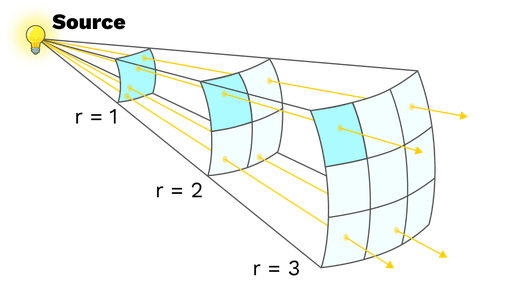An inverse-square law is any law that says that a quantity decreases with the inverse square of the distance from its source. Inverse-square laws are common because they show up everywhere where a source emits evenly in all directions. Examples of inverse-square laws are Newton’s universal law of gravitation, Coulomb’s law, or the law that describes the intensity of light irradiating from a source.

The case of the light source is the easiest to visualize. Imagine a lightbulb that emits light rays evenly in every direction. How many rays per unit area reach us? If we stand a meter away from the lightbulb, the light rays that reach us are spread out on the surface of a one-meter radius sphere. Yet, if we stand two meters away, the same quantity of rays are spread out on the surface of a sphere with a two-meter radius. Because the area of the sphere depends on the square of its radius (the surface S of a sphere of radius r is S=4πr2), the number of rays per unit area that reach us is one-fourth of the number of rays that reached us in the smaller one-meter sphere:

Rays per area in the 1m-radius sphere: Total rays/(4πr2 1-meter sphere)=Total ray/(4π12)=Total rays/(4π)

Rays per area in the 2m-radius sphere: Total rays/(4πr22-meter sphere)=Total rays/(4π22)=Total rays/(4π·4)

Thus:

Rays per area in the 2m-radius sphere = Rays per area in the 1m-radius sphere/4.

In general, for a sphere of radius r:

Rays per area in the r-radius sphere= Rays per area in the 1m-radius sphere/r2.

So the number of rays that reaches us is inversely proportional to the square of our distance to the lightbulb: we have an inverse-square law.

A similar line of reasoning is valid for the gravitational and electrostatic forces if we consider masses and charges as sources of field lines or, more accurately, of the gravitational and electrostatic fields.Figure: The further from the source, the bigger the sphere. The bigger the sphere, the bigger its area. The bigger area for the same amount of rays, the fewer rays per area. Because the area grows with the distance squared, the number of rays per unit area follows an inverse-square law.